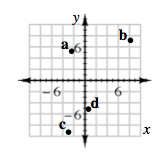### Home > CC2 > Chapter 2 > Lesson 2.2.6 > Problem2-105

2-105.

Draw a coordinate grid. Make both the $x\text{-axis}$ and $y\text{-axis}$ go from $-10$ to $10$. Then plot the following points. Label each point with its letter.

1. $(-2.2,4.64)$

1. $(8.2,7.59)$

1. $\left(-3\frac{4}{7},-9\frac{3}{10}\right)$

1. $(0.62,-5.1)$

Plot the points on the graph you made. Remember that the first point is the x-coordinate and the second point is the y-coordinate.